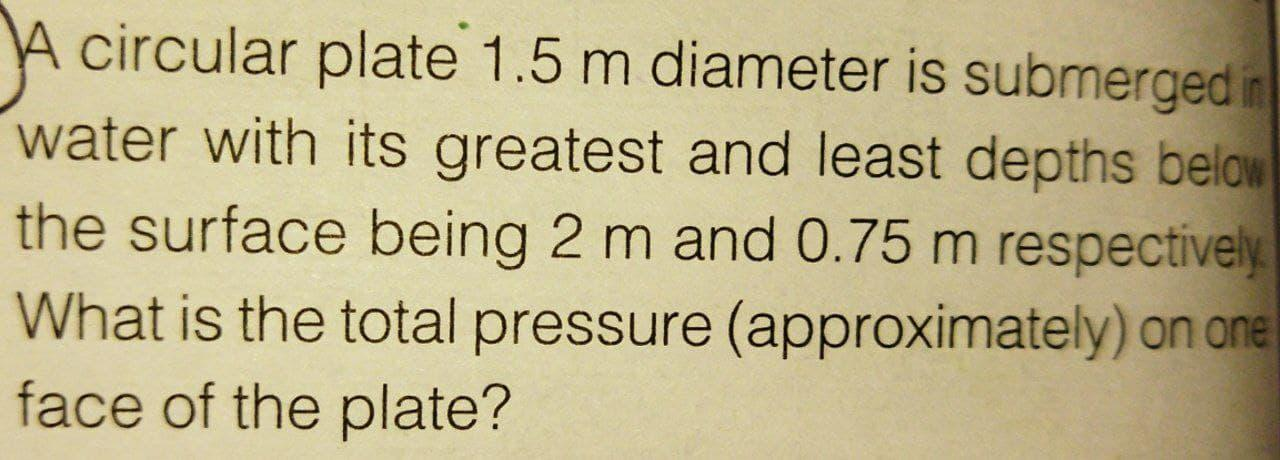# A circular plate 1.5 m diameter is submerged in water with its greatest and least depths below the surface being 2 m and 0.75 m respectively What is the total pressure (approximately) on one face of the plate?

Question-AnswerCategory: Fluid MechanicsA circular plate 1.5 m diameter is submerged in water with its greatest and least depths below the surface being 2 m and 0.75 m respectively What is the total pressure (approximately) on one face of the plate?

A circular plate 1.5 m diameter is submerged in water with its greatest and least depths below the surface being 2 m and 0.75 m respectively What is the total pressure (approximately) on one face of the plate?